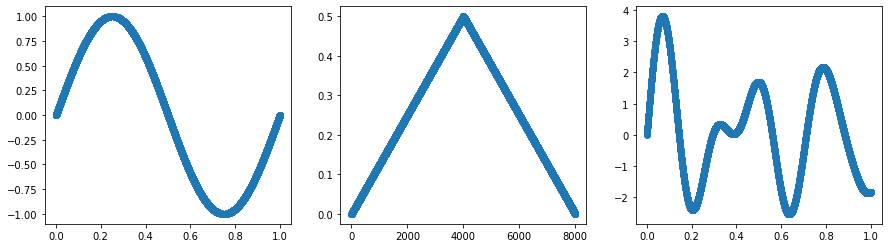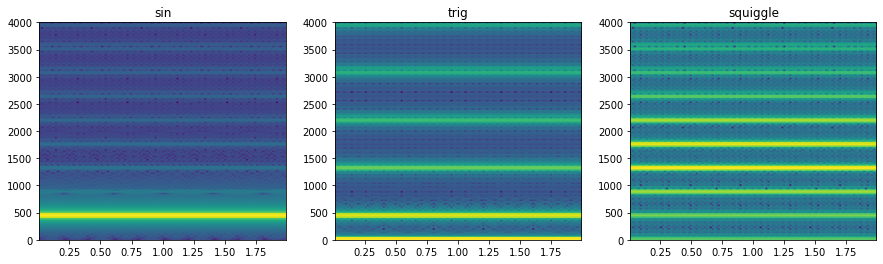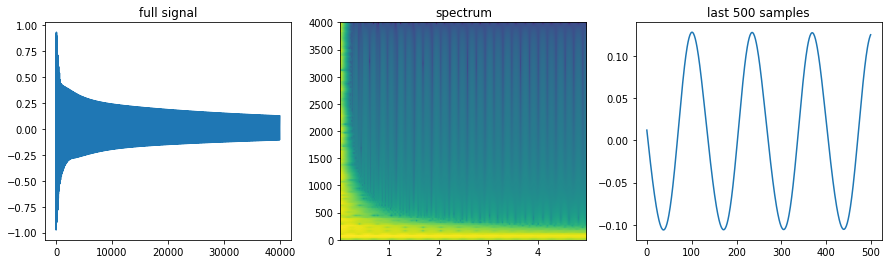# Interfacing a Synchronous Language withPython (and Jupyter Notebooks)

Guillaume Baudart (Inria Paris),
Louis Mandel (IBM Research, USA)

# I: High-Level Interactions¶

• A new Python backend for Zelus
• Compile a class for each node with two methods reset and step
• Nodes execution can interact with Python libraries
In :
import pyzls

In :
%%zelus -clear

let node nat(i) = o where
rec o = 0 fby o + i


Compiled code:

class nat(Node):
def __init__ (self):
self.m_10 = 42

def reset (self, ):
self.m_10 = 0

def step (self, i_8):
x_11 = self.m_10
self.m_10 = o_9
return o_9


To run a zelus node:

1. instantiate the class
2. call the reset method to initialize the memories
3. fire the step method as many times as you want
In :
n = nat()
n.reset()
[n.step(1) for _ in range(10)]

Out:
[1, 2, 3, 4, 5, 6, 7, 8, 9, 10]

## Example: Sound Synthesis in Pyzls¶

Inspiration: blog post https://flothesof.github.io/Karplus-Strong-algorithm-Python.html

In :
import numpy as np
import matplotlib.pyplot as plt
from IPython.display import Audio, display


### A simple buffer library based on numpy¶

• noise returns a buffer randomly filled with -1, and 1.
• freq_to_size turns a frequency into a buffer size (wavetable synthesis)
In :
@pyzls.lib("buffer", clear=True)
def noise(n: int) -> "'buff":
import numpy as np
return (2 * np.random.randint(0, 2, n) - 1).astype(np.float)

@pyzls.lib("buffer")
def get(b:"'buff", i:int) -> "'a":
return b[i]

@pyzls.lib("buffer")
def update(b:"'buff" , i:int, v:"'a") -> "unit":
b[i] = v

@pyzls.lib("buffer")
def size(b: "'buff") -> int:
return len(b)

@pyzls.lib("buffer")
def freq_to_size(f: float, fs: int) -> int:
return int(fs // f)


### Wavetable synthesis¶

• use a ring buffer as a lookup table
• sound is produced by repeatedly reading the buffer which produces a periodic signal
In :
%%zelus -clear

let fs = 8000

open Buffer

let node wavetable (b, speed) = y where
rec i = 0 fby (i+speed) mod size(b)
and y = get(b, i)


Let's try different shapes.

In :
t = np.linspace(0, 1, num=fs)
table_sin = np.sin(2 * np.pi * t)
table_trig = t * (t < 0.5) + (1 - t) * (t > 0.5)
table_squiggle = np.sum([np.sin(2 * np.pi * t * f)
for f in np.random.rand(4) * 5],
axis=0)

fig, axes = plt.subplots(nrows=1, ncols=3, figsize=(15, 4))
axes.plot(t, table_sin, '-o')
axes.plot(table_trig, "-o")
axes.plot(t, table_squiggle, '-o')

Out:
[<matplotlib.lines.Line2D at 0x11483f430>]Let's run our wavetable node with a simple sinus.

In :
w = wavetable()
w.reset()
samples_sin = [w.step(table_sin, 440) for _ in range(2*fs)]


We can also reset and run the node with new inputs

In :
w.reset()
samples_trig = [w.step(table_trig, 440) for _ in range(2*fs)]
w.reset()
samples_squiggle = [w.step(table_squiggle, 440) for _ in range(2*fs)]


Let's hear the results...

In :
display(Audio(samples_sin, rate=fs))
display(Audio(samples_trig, rate=fs))
display(Audio(samples_squiggle, rate=fs))


Complexity of the wavetable is reflected in the spectrum

In :
fig, axes = plt.subplots(nrows=1, ncols=3, figsize=(15, 4))
axes.set_title("sin")
_ = axes.specgram(samples_sin, Fs=fs)
axes.set_title("trig")
_ = axes.specgram(samples_trig, Fs=fs)
axes.set_title("squiggle")
_ = axes.specgram(samples_squiggle, Fs=fs)### Karplus-Strong synthesis¶

Digital Synthesis of Plucked-String and Drum Timbres K. Karplus and A. Strong Computer Music Journal, Vol. 7, No. 2 (Summer, 1983), pp. 43-55

The wavetable-synthesis technique is very simple but rather dull musically, since it produces purely periodic tones. Traditional musical instruments produce sounds that vary with time. This variation can be achieved in many ways on computers. The approach in FM synthesis, additive synthesis, subtractive synthesis, and waveshaping is to do further processing of the samples after taking them from the wavetable. All the algorithms described in this paper produce the variation in sound by modifying the wavetable itself.

The Karplus-Strong algorithm is a variation of wavetable synthesis where the ring buffer intialized with noise and dynamically updated with the following formula:

$Y_t = \frac{1}{2} (Y_{t−p} + Y_{t−p−1})$In :
%%zelus

open Buffer

let node karplus_strong(f) = y where
rec init n = freq_to_size(f, fs)
and init b = noise(n)
and i = 0 fby (i+1) mod n
and y = 0.5 *. (get(b, i) +. 0.0 fby y)
and _ = update(b, i, y)

In :
kp = karplus_strong()
kp.reset()
samples = [kp.step(60) for _ in range(5*fs)]


Here we get the signal of a pinched string at 220Hz (A).

In :
fig, axes = plt.subplots(nrows=1, ncols=3, figsize=(15, 4))
axes.set_title("full signal")
axes.plot(samples)
axes.set_title("spectrum")
_ = axes.specgram(samples, Fs=fs)
axes.set_title("last 500 samples")
axes.plot(samples[-500:])

Out:
[<matplotlib.lines.Line2D at 0x114db6d00>]In :
Audio(samples, rate=fs)

Out:

### Simple player using parameterized states¶

In state Play(f):

• pinch a string with frequency $f$
• wait 1s
• restart state Play with a new frequency $f*\sqrt{2}^{12}$ (one semitone higher with equal temperament)
In :
%%zelus

let node seconds() = s where
rec t = (0 fby t + 1) mod fs
and s = (0 fby s) + if t = 0 then 1 else 0

let node scale() = o where
rec automaton
| Play(f) -> do  s = seconds()
and o = karplus_strong(f)
until (s = 1) then Play(f *. 1.05946)
init Play(65.)

In :
s = scale()
s.reset()
samples = [s.step(_) for _ in range(8*fs)]
Audio(samples, rate=fs)

Out: